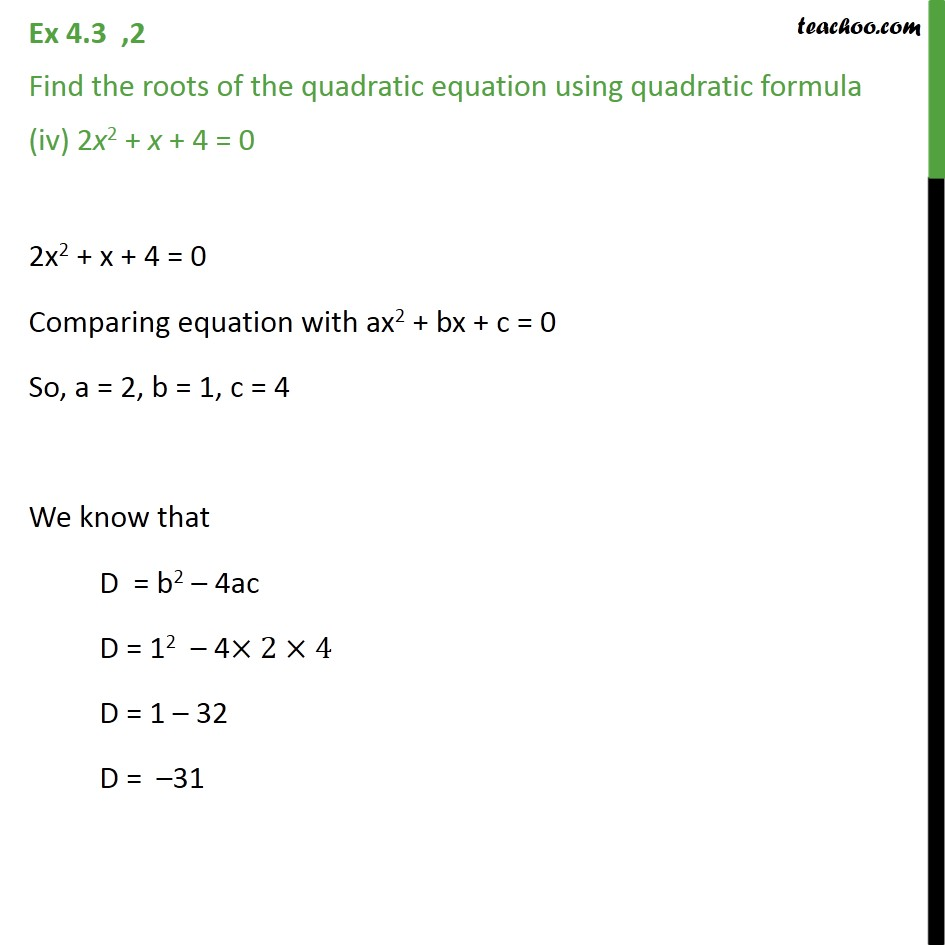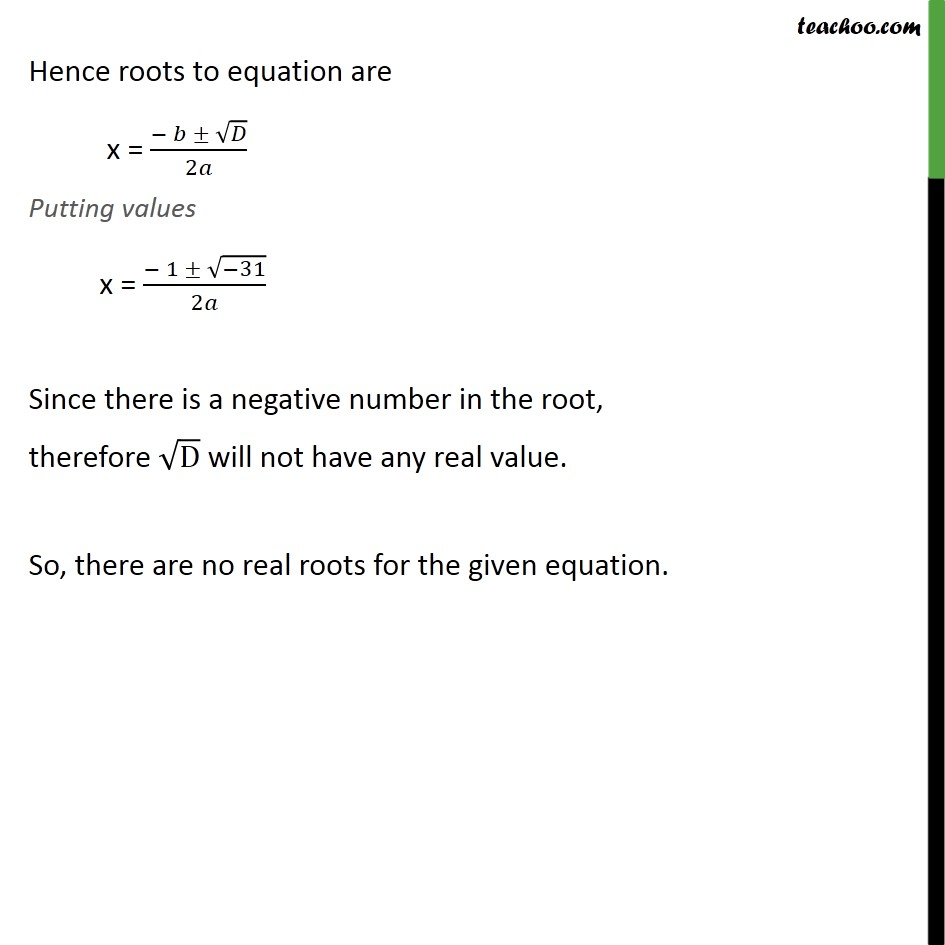Ex 4.3

Chapter 4 Class 10 Quadratic Equations
Serial order wiseLearn in your speed, with individual attention - Teachoo Maths 1-on-1 Class

### Transcript

Ex 4.3 ,2 Find the roots of the quadratic equation using quadratic formula (iv) 2x2 + x + 4 = 0 2x2 + x + 4 = 0 Comparing equation with ax2 + bx + c = 0 So, a = 2, b = 1, c = 4 We know that D = b2 4ac D = 12 4 2 4 D = 1 32 D = 31 Hence roots to equation are x = ( )/2 Putting values x = ( 1 ( 31))/2 Since there is a negative number in the root, therefore D will not have any real value. So, there are no real roots for the given equation.